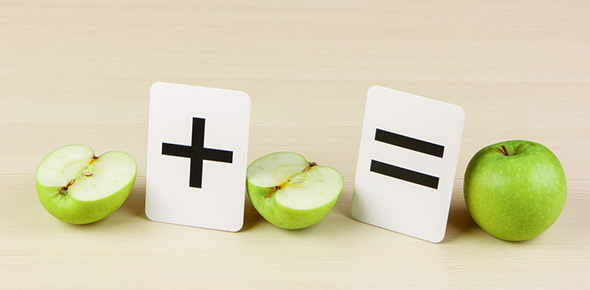14 Questions | Total Attempts: 148SettingsThis quiz will assess and reinforce the student's ability to interpret and calculate equivalent fractions properly and efficiently. It can also be used as a preparatory tool for an upcoming exam or a test.

• 1.
A fraction is an expression of a division operation
• A.

True

• B.

False

• 2.
Each number can be expressed as the product of two or more
• A.

Whole numbers

• B.

Decimal numbers

• C.

Prime Numbers

• 3.
For any fraction, higher equivalent fractions can be calculated by
• A.

Multiplying numerator and denominator by the same integer

• B.

Multiplying numerator and denominator by different integers

• C.

Dividing numerator and denominator by the same integer

• 4.
When dividing numerator by denominator, equivalent fractions will always give
• A.

The same value

• B.

The same spelling mistake

• C.

The same decimal

• 5.
4/7 and 16/27 are equivalent fractions
• A.

True

• B.

False

• 6.
3/8 and 18/40 are equivalent fractions
• A.

True

• B.

False

• 7.
7/11 and 56/88 are equivalent fractions
• A.

True

• B.

False

• 8.
Which fraction is not equivalent?
• A.

14/34

• B.

35/56

• C.

5/8

• 9.
Which fraction is not equivalent?
• A.

3/5

• B.

18/30

• C.

15/20

• 10.
Which fraction is not equivalent?
• A.

30/50

• B.

15/25

• C.

5/9

• 11.
What fractions are equivalent to 12/30?  (there may be more than one!)
• A.

24/60

• B.

2/5

• C.

4/11

• 12.
What fractions are equivalent to 3/7?  (there may be more than one!)
• A.

21/49

• B.

42/98

• C.

15/35

• 13.
What fractions are equivalent to 31/55?  (there may be more than one!)
• A.

3/6

• B.

6/10

• C.

62/111

• D.

None

• 14.
What fractions are equivalent to 2.45?  (there may be more than one!)
• A.

245/100

• B.

2.45/100

• C.

49/20

Related TopicsBack to top